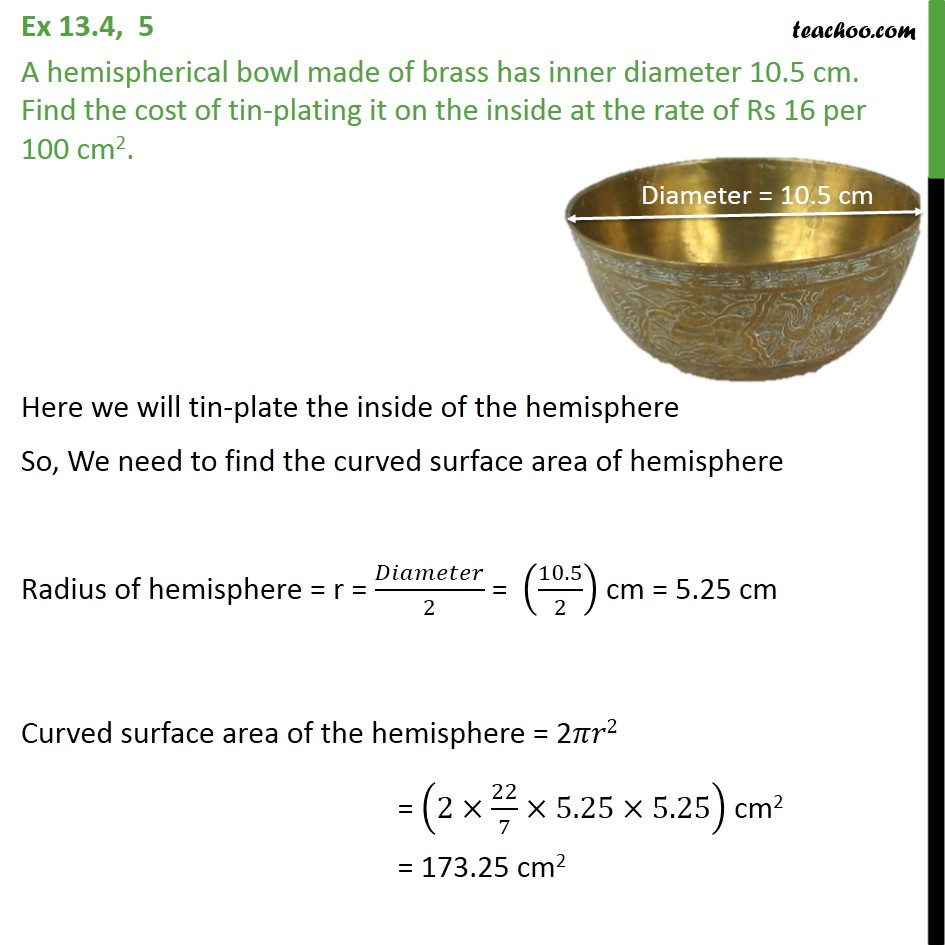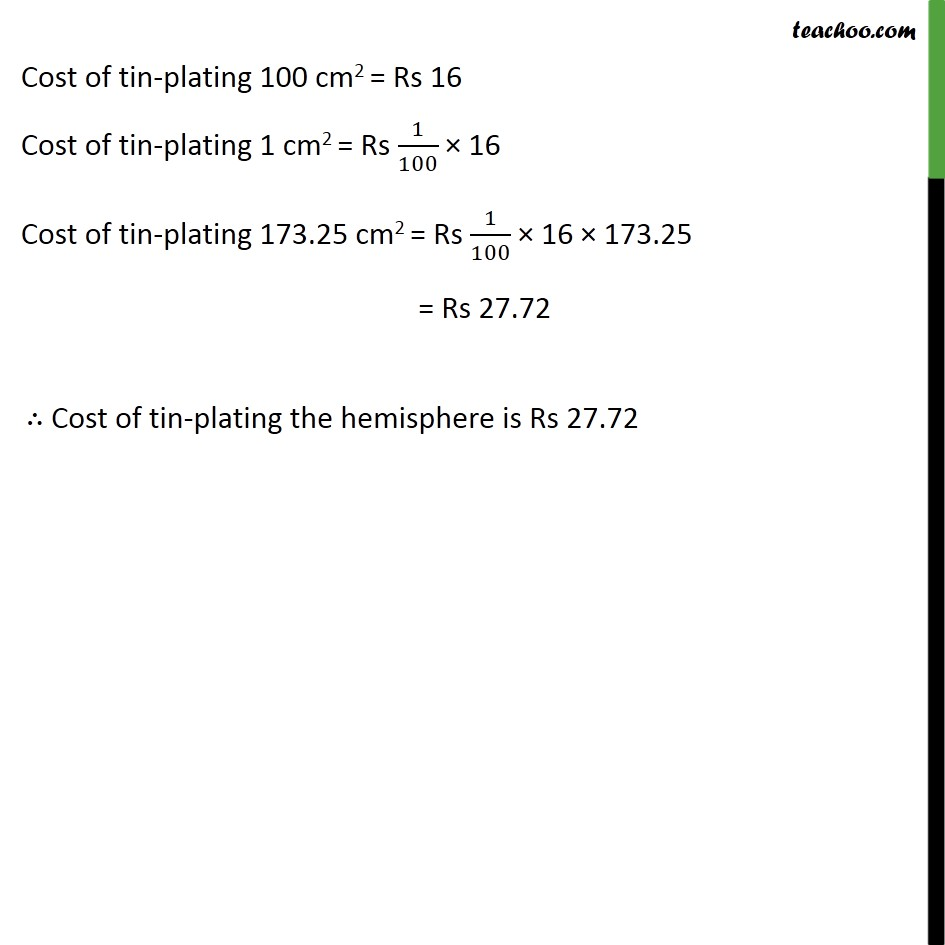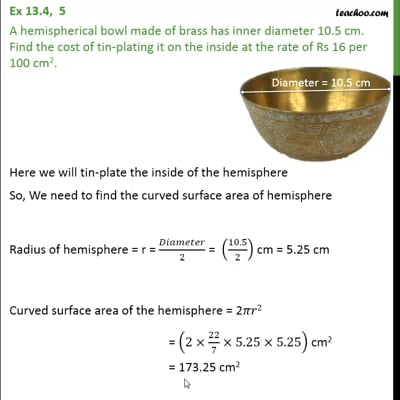Area Of Hemisphere

Chapter 13 Class 9 Surface Areas and Volumes
Concept wiseThis video is only available for Teachoo black users

Introducing your new favourite teacher - Teachoo Black, at only ₹83 per month

### Transcript

Ex 13.4, 5 A hemispherical bowl made of brass has inner diameter 10.5 cm. Find the cost of tin-plating it on the inside at the rate of Rs 16 per 100 cm2. Here we will tin-plate the inside of the hemisphere So, We need to find the curved surface area of hemisphere Radius of hemisphere = r = /2 = (10.5/2) cm = 5.25 cm Curved surface area of the hemisphere = 2 2 = (2 22/7 5.25 5.25) cm2 = 173.25 cm2 Cost of tin-plating 100 cm2 = Rs 16 Cost of tin-plating 1 cm2 = Rs 1/100 16 Cost of tin-plating 173.25 cm2 = Rs 1/100 16 173.25 = Rs 27.72 Cost of tin-plating the hemisphere is Rs 27.72